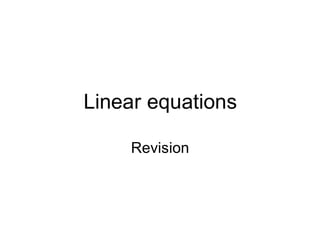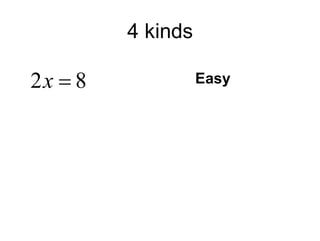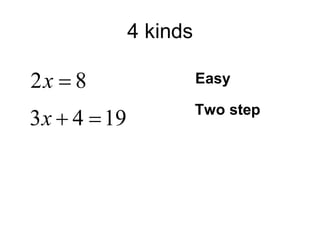Successfully reported this slideshow.

# Linear Equations Slide Share Version Exploded

9

Share×
1 of 81
1 of 81

# Linear Equations Slide Share Version Exploded

9

Share

GCSE Maths algebra linear equations revision, now tested by students and typos eliminated. Simple, two step, x on each side and bracket type equations but all examples have whole number answers.

GCSE Maths algebra linear equations revision, now tested by students and typos eliminated. Simple, two step, x on each side and bracket type equations but all examples have whole number answers.

## More Related Content

### Related Books

Free with a 14 day trial from Scribd

See all

### Related Audiobooks

Free with a 14 day trial from Scribd

See all

### Linear Equations Slide Share Version Exploded

1. 1. Linear equations Revision
2. 2. 4 kinds Easy
3. 3. 4 kinds Easy Two step
4. 4. 4 kinds Easy Two step X on both sides
5. 5. 4 kinds Easy Two step X on both sides Brackets
6. 6. Easy examples
7. 7. Easy examples
8. 8. Easy examples What is the 3 doing?
9. 9. Easy examples What is the 3 doing? Multiplying the x
10. 10. Easy examples What is the 3 doing? Multiplying the x
11. 11. Easy examples What is the 3 doing? Multiplying the x Do the opposite!
12. 12. Easy examples What is the 3 doing? Multiplying the x Do the opposite! Divide by 3
13. 13. Easy examples What is the 3 doing? Multiplying the x Do the opposite! Divide by 3
14. 14. Easy examples
15. 15. Easy examples
16. 16. Easy examples What is the 6 doing?
17. 17. Easy examples What is the 6 doing? Adding to the x
18. 18. Easy examples What is the 6 doing? Adding to the x Do the opposite!
19. 19. Easy examples What is the 6 doing? Adding to the x Do the opposite! Subtract 6
20. 20. Easy examples What is the 6 doing? Adding to the x Do the opposite! Subtract 6
21. 21. Easy examples
22. 22. Easy examples
23. 23. Easy examples What is the 4 doing?
24. 24. Easy examples What is the 4 doing? Dividing the x
25. 25. Easy examples What is the 4 doing? Dividing the x Do the opposite!
26. 26. Easy examples What is the 4 doing? Dividing the x Do the opposite! Multiply by 4
27. 27. Easy examples What is the 4 doing? Dividing the x Do the opposite! Multiply by 4
29. 29. Your turn X=32 X=4 X=9
30. 30. Two step equations
31. 31. Two step equations
32. 32. Two step equations Undo the +4
33. 33. Two step equations Undo the +4
34. 34. Two step equations Undo the +4 Undo the x3
35. 35. Two step equations Undo the +4 Undo the x3
36. 36. Two step equations
37. 37. Two step equations
38. 38. Two step equations Undo the -3
39. 39. Two step equations Undo the -3
40. 40. Two step equations Undo the -3 Undo the x2
41. 41. Two step equations Undo the -3 Undo the x2
43. 43. Your turn X = 5 X = 6 X = 4
44. 44. X on both sides
45. 45. X on both sides
46. 46. X on both sides Take 3x off both sides
47. 47. X on both sides Take 3x off both sides
48. 48. X on both sides Take 3x off both sides Undo -4
49. 49. X on both sides Take 3x off both sides Undo -4
50. 50. X on both sides Take 3x off both sides Undo -4 Undo x2
51. 51. X on both sides Take 3x off both sides Undo -4 Undo x2
52. 52. X on both sides
53. 53. X on both sides
54. 54. X on both sides Add 2x to both sides
55. 55. X on both sides Add 2x to both sides
56. 56. X on both sides Add 2x to both sides Undo +6
57. 57. X on both sides Add 2x to both sides Undo +6
58. 58. X on both sides Add 2x to both sides Undo +6 Undo x5
59. 59. X on both sides Add 2x to both sides Undo +6 Undo x5
61. 61. Your turn X = 7 X = 3 X = 5 X = 10
62. 62. Brackets
63. 63. Brackets
64. 64. Brackets Multiply out bracket
65. 65. Brackets Multiply out bracket
66. 66. Brackets Multiply out bracket Collect terms
67. 67. Brackets Multiply out bracket Collect terms
68. 68. Brackets Multiply out bracket Collect terms Undo +6
69. 69. Brackets Multiply out bracket Collect terms Undo +6
70. 70. Brackets Multiply out bracket Collect terms Undo +6 Undo x7
71. 71. Brackets Multiply out bracket Collect terms Undo +6 Undo x7
72. 72. Brackets
73. 73. Brackets
74. 74. Brackets
75. 75. Brackets
76. 76. Brackets
77. 77. Brackets